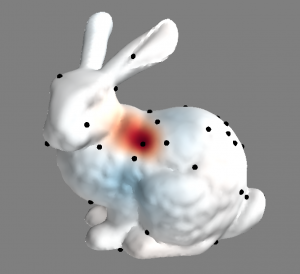## A New Method to Approximate Symmetric Matrices

Symmetric matrices are very common in many fields. For example, they are used in Kernel Machines to  maintain pairwise kernel functions, while in computer vision they represent pairwise distances between points. When a dataset contains ten thousands or more points, these symmetric matrices do not fit in memory and may be too expensive to compute. Existing alternatives suggest to approximate this matrix using a low-rank approximation. Nyström is a very powerful method that samples a subset of the data points and uses them to approximate the matrix. Many research has centered around theory, sampling schemes, and accuracy improvements. However, the method suffers from some practical limitations: it may become unstable when the matrix is not positive semidefinite, and when applied to millions of data points the memory requirements growth, limiting the accuracy of the method.

With Professor Alex Huth, we developed a new method to approximate symmetric matrices that overcomes these limitations. We dubbed our method biharmonic matrix approximation (BHA). Assuming the data points reside in a manifold, BHA samples a subset of data points and interpolates the manifold from this subset using Biharmonic interpolation. The method computes the symmetric matrix of this sampled subset and utilizes the interpolation to approximate the results to the other points. This means of approximation avoids numerical instabilities that exists in other methods. Moreover, the interpolation construction of BHA assigns higher weights to nearby sampled point than to those farther away. From this observation, we construct a sparse version of BHA that reduces the memory consumption enabling approximation of millions of data points and similar accuracy.The figure depicts the weights for the black vertex in the red zone. The weights are over all the other vertices on the mesh. Red symbolizes positive values, blue negative values and white a near-zero value. This weights represent a column of the interpolation operator of BHA.